Next: Hollow cylinder, transient 1-D. Up: Cylindrical Coordinates. Transient 1-D. Previous: Infinite body with circular

### Solid cylinder transient 1-D.

R01 Solid cylinder 0 < r < b, with G = 0 (Dirichlet) at r = b.
 GR01(r, t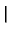r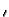,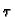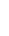) =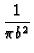exp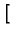-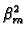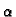(t -)/b2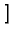x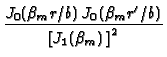with eigenvalues given by J0(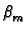) = 0.

R02 Solid cylinder 0 < r < b, with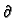G/r = 0 (Neumann) at r = b.

 GR02(r, tr,) =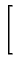1 +exp-(t -)/b2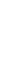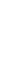x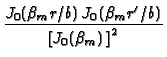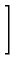with eigenvalues given by J1() = 0.

R03 Solid cylinder 0 < r < b, with kG/r + hG = 0 (convection) at r = b.

 GR03(r, tr,) =exp-(t -)/b2x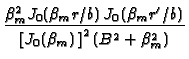with eigenvalues given by -J1() + BJ0() = 0 , and B = hb/k.Next: Hollow cylinder, transient 1-D. Up: Cylindrical Coordinates. Transient 1-D. Previous: Infinite body with circular
Kevin D. Cole
2002-12-31